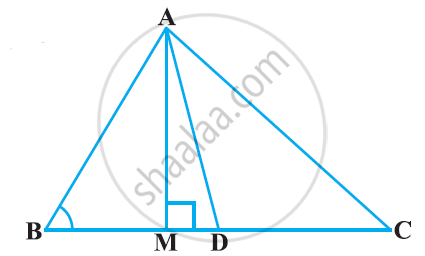# In the given figure, AD is a median of a triangle ABC and AM ⊥ BC. Prove that: - Mathematics

In the given figure, AD is a median of a triangle ABC and AM ⊥ BC. Prove that:1)AC^2 = AD^2 + BC . DM + ((BC)/2)^2

2) AB^2 = AD^2 – BC . DM + ((BC)/2)^2

3) AC^2 + AB^2 = 2 AD^2 + 1/2(BC)^2

#### Solution

(i) Applying Pythagoras theorem in ΔAMD, we obtain

AM2 + MD2 = AD2 … (1)

Applying Pythagoras theorem in ΔAMC, we obtain

AM2 + MC2 = AC2

AM2 + (MD + DC)2 = AC2

(AM2 + MD2) + DC2 + 2MD.DC = AC2

AD2 + DC2 + 2MD.DC = AC2 [Using equation (1)]

Using the result, DC = BC/2, we obtain

AD^2+((BC)/2)^2 + 2MD.((BC)/2)= AC^2

AD^2+((BC)/2)^2 + MC xx BC = AC^2

(ii) Applying Pythagoras theorem in ΔABM, we obtain

AB2 = AM2 + MB2

= (AD2 − DM2) + MB2

= (AD2 − DM2) + (BD − MD)2

= AD2 − DM2 + BD2 + MD2 − 2BD × MD

= AD2 + BD2 − 2BD × MD

= AD^2+((BC)/2)^2 - 2((BC)/2) xx MD

= AD^2 + (

(iii)Applying Pythagoras theorem in ΔABM, we obtain

AM2 + MB2 = AB2 … (1)

Applying Pythagoras theorem in ΔAMC, we obtain

AM2 + MC2 = AC2 … (2)

Adding equations (1) and (2), we obtain

2AM2 + MB2 + MC2 = AB2 + AC2

2AM2 + (BD − DM)2 + (MD + DC)2 = AB2 + AC2

2AM2+BD2 + DM2 − 2BD.DM + MD2 + DC2 + 2MD.DC = AB+ AC2

2AM2 + 2MD2 + BD2 + DC2 + 2MD (− BD + DC) = AB2 + AC2

2(AM^2 + MD^2) + ((BC)/2)^2 + ((BC)/2)^2 + 2MD ((-BC)/2 + (BC)/2) = AB^2+AC^2

2AD^2 + (BC^2)/2 = AB^2 + AC^2

Concept: Right-angled Triangles and Pythagoras Property
Is there an error in this question or solution?

#### APPEARS IN

NCERT Class 10 Maths
Chapter 6 Triangles
Exercise 6.6 | Q 5 | Page 152